# AP Chemistry : Reaction Types

## Example Questions

### Example Question #2 : Fundamental Reaction Concepts

What is the balanced chemical equation for the combustion of butane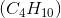?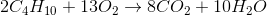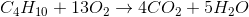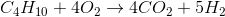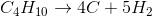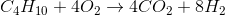Explanation:

Combustion is the chemical reaction of a hydrocarbon with molecular oxygen, and it always produces carbon dioxide and water. Knowing the reactants and products, the unbalanced equation must be: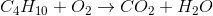We start by balancing the hydrogens. Since there are 10 on the left and only 2 on the right, we put a coefficient of 5 on water.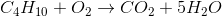Similarly, we balance carbons by putting a 4 on the carbon dioxide.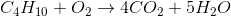To find the number of oxygens on the right, we multiply the 4 coefficient by the 2 subscript on O (which gets us 8 oxygens) and then add the 5 oxygens from the 5 water molecules to get a total of 13. The needed coefficient for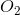on the left would then have to be 13/2.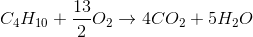Because fractional coefficients are not allowed, we mutiply every coefficient by 2 to find our final reaction: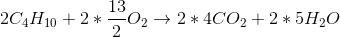### Example Question #1 : Balancing Chemical Equations

Determine whether or not solid aluminum reacts with aqueous zinc chloride. If it does, determine the balanced equation for the reaction.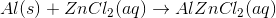No reaction occurs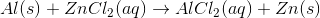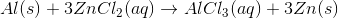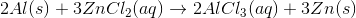Explanation:

When we check the activity series, it is fairly easy to see that aluminum metal is more reactive than zinc metal. So, in this case, the two metals undergo a redox reaction, where the aqueous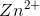is reduced to solid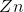, and the solid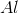is oxidized to aqueous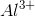. These charges are the common oxidation states for zinc and aluminum and should be memorized.

Becauseis the new species, it bonds with 3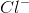ions. The unbalanced equation is: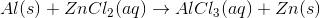We note that there are 2 chlorine atoms on the left and 3 chlorine atoms on the right. To balance, we use a 3 coefficient on the left and a 2 coefficient on the right. This gives a total of 6 chlorine atoms on eahc side.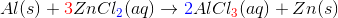However, now we have also increased the amounts of zinc and aluminum. We copy the necessary coefficients to balance those—2 for aluminum on the left, 3 for zinc on the right—and we are done:### Example Question #1 : Balancing And Predicting Reactions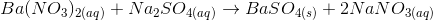What is the net ionic form of this equation?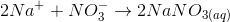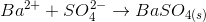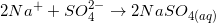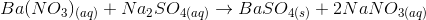Explanation:

To find the net ionic form of this chemical equation, first balance the equation, then dissociate all soluble components. In other words, separate the aqueous terms into two separate molecules/atoms. For example,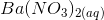dissociates into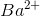and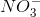. After separating all soluble terms, balance the equation and cancel out any terms that repeat on both sides of the equation. For example,will be on both sides of the equation after separating all terms so you can cross them out. Below are the steps taken to find the net ionic form of this chemical equation.Write out the dissociated forms of each species, if applicable.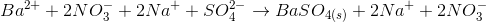Cross out species that appear on both sides of the equation. The net ionic equation is:### Example Question #2 : Balancing And Predicting Reactions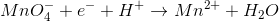What is the coefficient for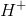, reduced to the lowest whole number, when the given reaction is balanced?Explanation:

The best way to solve this problem is to try all the possible answers. When we try 8,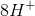means that there must be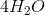means that there must be just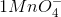, which means that there is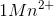. When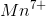is reduced to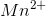, there are 5 remaining electrons. So 8 is correct. Alternatively, this question may be answered by the classical "trial and error" method of balancing equations.

### Example Question #1 : Single And Double Replacement Reactions

Given that lithium is higher on the activity series than hydrogen, what should be seen when solid lithium and hydrochloric acid are mixed?

None of these

Increase in test tube's temperature

Change in color

No change

Bubbles

Bubbles

Explanation:

Given that lithium is more reactive than hydrogen, expect a single replacement reaction to take place, so lithium will replace hydrogen.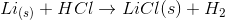All ionic compounds are solids at room temperature, which is why lithium chloride has the solid phase label. Remember that hydrogen is a diatomic element which is a gas a room temperature. When observing reactions in which gasses are produced from a solution, bubbles are seen. There is no indication whether this reaction is endothermic or exothermic, nor is there any information that suggests the reaction should change color.

### Example Question #1 : Single And Double Replacement Reactions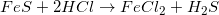What type of reaction is shown above?

Combustion

Single replacement

Decomposition

Double replacement

Double replacement

Explanation:

The typical double replacement reaction is as follows: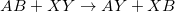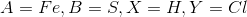The anions are the chemical species being exchanged (both sulfur and chloride). Hence, the name double replacement is given to this particular reaction.

### Example Question #2 : Single And Double Replacement Reactions

The following unbalanced reaction is important in the process of Steel Manufacturing: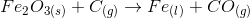Balance the following equation and name it's reaction type:, double replacement reaction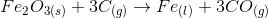, single replacement reaction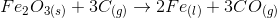, double replacement reaction, single displacement reaction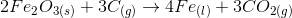, single replacement reaction, single displacement reaction

Explanation:

## Use the following steps to balance the equation:

1.) Check for diatomic molecules: in this case there are none so move on to step 2

2.) Balance the metals (hydrogen is not considered a metal in this application): There are 2 iron atoms on the left hand side and 1 iron atom on the right hand side so we add a 2 in front of the Fe on the right hand side to give: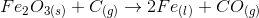3.) Balance the nonmetals (not including oxygen). There is one carbon on both sides so move on to step 4

4.) Balance oxygen. There are 3 oxygens on the left hand side and only 1 on the right hand side, so we can add a 3 in front of carbon monoxide on the right hand side to give: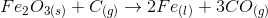5.) Balance hydrogen. No hydrogens, so we move on.

6.) Recount all atoms to make sure you have balanced correctly. There are 2 iron atoms on both sides and 3 oxygen on both sides.  However our carbons are no longer balanced so we can add a 3 in front of the elemental carbon on the left hand side to give:If this problem had involved ionic species you would also want to make certain that the charges are balanced on both sides of the equation.

The charges on both sides add up to zero so your equation is balanced:## Single v.s Double Replacement Reaction:

#### Single replacement Reaction:

A single replacement reaction is defined as a type of reduction-oxidation reaction in which a more active element replaces a less active element in a compound. These reactions produce a new compound and an element as the products.

General chemical equation: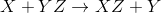#### Double Replacement Reaction:

A double replacement reaction is a type of reaction in which two reacting chemical species exchange bonds (ionic or covalent) resulting in the creation of two new products.

General chemical equation: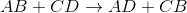is also important in steel manufacturing and is a single replacement reaction. However, the equation given wasso the difference being carbon monoxide production rather than carbon dioxide production. In reality both reactions occur simultaneously.

### Example Question #1 : Single And Double Replacement Reactions

What is used as guidelines to determine the phase labels for double displacement/double replacement reactions?

Solubility rules

Oxidation-reduction

Activity series

Huckel number

Octet rule

Solubility rules

Explanation:

For double displacement, the solubility rules determine the phase labels. Make sure the correct set of rules/guidelines for the type of reaction that is being written/balanced are used. The octet rule refers to the stability atoms achieve by filling their outer shell of electrons. Huckel number is 4n+2 where n is any integer, and is involved in assigning aromaticity.

### Example Question #5 : Single And Double Replacement Reactions

For single replacement reactions, which of the following sets of guidelines are most helpful?

Oxidation-reduction

Boyle's law

Solubility rules

Activity series

Octet rule

Activity series

Explanation:

When writing/balancing a single replacement reaction, make sure to consult the activity series, because that will tell us if a reaction will actually take place. Remember to look at the cations (metals and hydrogen) and where they are in relation to each other on the activity series. The higher one on the activity series is the most reactive; so if the cation by itself is the most reactive, it will replace the other cation and a reaction will occur. Boyle's law explains the inverse relationship between volume and pressure with respect to certain amount of an ideal gas at constant temperature.

### Example Question #1 : Balancing Oxidation Reduction Reactions

The following ReDox reaction takes place in acidic solution:

Fe2+ + Cr2O72– → Fe3+ + Cr3+

What is the sum of coefficients in this redox reaction?

36

34

35

33

37